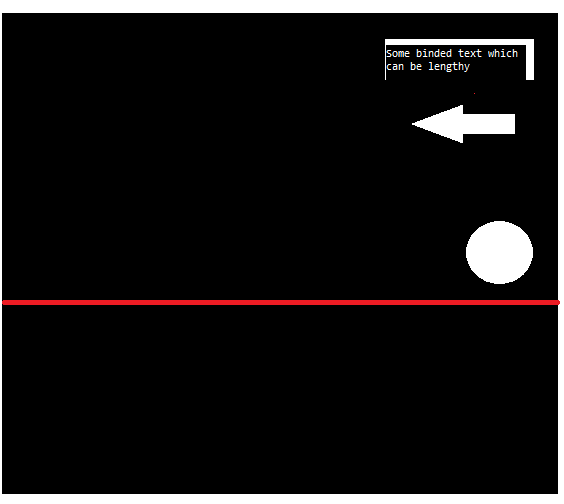# Xamarin Forms : How to place a view inside a relative layout, starting at the right end and expandin

I have a design requirement as in image(although this is the simplified version).

I need the end x-coordinates of the button and the image to align and the button needs to expand towards left, depending upon the binded text.

What I have done:

```<RelativeLayout Padding="0,0,0,0" Margin="0,0,0,0"
VerticalOptions="FillAndExpand"
HorizontalOptions="FillAndExpand">

//other children

<Image Source="circle.png"
Aspect="AspectFit"
x:Name="circleImg"
RelativeLayout.YConstraint="{ConstraintExpression Type=RelativeToParent,
Property=Width, Factor=0.48, Constant=0}"
RelativeLayout.XConstraint="{ConstraintExpression Type=RelativeToParent,
Property=Width, Factor=0.811, Constant=0}"
RelativeLayout.WidthConstraint="{ConstraintExpression Type=RelativeToParent,Property=Width, Factor=0.1593, Constant = 0}"
RelativeLayout.HeightConstraint="{ConstraintExpression Type=RelativeToParent,Property=Width, Factor=0.1593, Constant = 0}">
</Image>

<Button
Text="{Binding ButtonText!}"
TextColor="White"
BorderColor="Transparent"
RelativeLayout.YConstraint="{ConstraintExpression Type=RelativeToParent,
Property=Height, Factor=0.0, Constant=0}"
RelativeLayout.XConstraint="{ConstraintExpression Type=RelativeToView,
ElementName=circleImg, Property=X, Factor=1, Constant=0}"
RelativeLayout.WidthConstraint="{ConstraintExpression Type=RelativeToParent,Property=Width, Factor=0.1593, Constant = 0}"/>

//other children

</RelativeLayout>
```

This gives me a button with start aligned with the image's start position, and with width equal to the image. However, if the binded text is long, the text gets truncated.
I tried `VerticalOptions="EndAndExpand"` , but it doesn't work.

Is there a way I can specify the ending x-coordinate of the button, rather than its start, and make it expand towards left?Tagged: